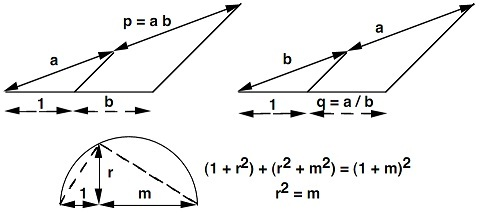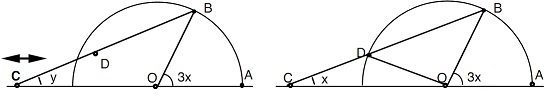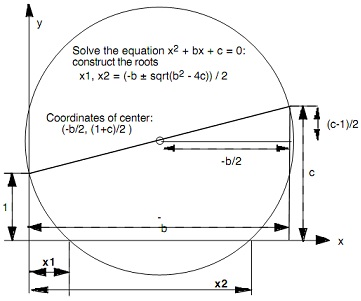#### Concept of Construction with ruler and compass, Models of Computation

Construction with ruler and compass:

Plato (427-347 B.C.) considered ruler and compass the merely appropriate tools for geometric construction.

A) Primitive objects: straight line (finite or unbounded), point and circle.

B) Primitive operations:

•    Given 2 points P, Q construct a line passing via P and Q
•    Given 2 points P, Q construct a circle with center P which passes via Q
•    Given 2 lines L, L’and two circles C, C’ or a line L and a circle C,
•    Make a new point P at any intersection of such a pair.

C) Compound object: Any structure built from the primitive objects by following a series of primitive operations.

Illustrations:

A) Given a line L and a point P on L, then construct a line L’ passing via P and orthogonal to the L.

B) Construct a pair of Lines L, L’ which defines angles of 90º, 60º, 45º and 30º respectively.

C) The segment is a triple (L, P, Q) with points P and Q on L. The segment of length 1 might be given or it might be randomly constructed to define the unit of measurement.

The angles and lengths which can be constructed:

A) Beginning with given angles, construct additional angles through bisection, subtraction and addition.

B) Beginning with a segment of unit length, construct the additional lengths through bisection, addition, subtraction, division, multiplication and square root.Therefore, construction by ruler and compass is reduced the question whether certain desired quantity can be stated in terms of rational operations and square roots.

Theorem: The proposed construction is probable by ruler and compass if the numbers that define analytically the preferred geometric elements can be derived from such defining the given elements by the finite number of rational operations and extractions of the real square roots.

Possible construction: Equilateral polygon of 17 sides, ‘17-gon’ (that is, Carl Friedrich Gauss 1777-1855)

Impossible constructions: Equilateral heptagon, ‘7-gon’ (Gauss). Doubling the unit cube (simplify x3 = 2).

Theorem: There is no algorithm to trisect the arbitrary angle with the ruler and compass. And yet, the Archimedes (~287 B.C. - ~ 212 B.C) found a process to trisect the arbitrary angle:

Given any angle AOB = 3x in a unit circle. The ruler CB has a mark D at distance CD = radius = r = 1. Slide C all along the x-axis till D lies on circle. Then, angle ACB = x.Msg: Minor modifications in a model of computation might have drastic consequences- precise definition required!

Example: The quadratic equation x2 + bx + c = 0 consists of roots x1, x2 = (-b ± sqrt(b2 - 4c))/2. Now prove that in the ruler and compass construction illustrated below, the segments x1 and x2 are solutions of this equation.Solution: Replace xc = - b/2 and yc = (1 + c)/2 in the circle equation (x - xc)2 + (y - yc)2, set y = 0  to get the quadratic equation  x2 + bx + c = 0 whose roots are the preferred values  x1 and x2 .

Latest technology based Theory of Computation Online Tutoring Assistance

Tutors, at the www.tutorsglobe.com, take pledge to provide full satisfaction and assurance in Theory of Computation help via online tutoring. Students are getting 100% satisfaction by online tutors across the globe. Here you can get homework help for Theory of Computation, project ideas and tutorials. We provide email based Theory of Computation help. You can join us to ask queries 24x7 with live, experienced and qualified online tutors specialized in Theory of Computation. Through Online Tutoring, you would be able to complete your homework or assignments at your home. Tutors at the TutorsGlobe are committed to provide the best quality online tutoring assistance for Theory of Computation Homework help and assignment help services. They use their experience, as they have solved thousands of the Theory of Computation assignments, which may help you to solve your complex issues of Theory of Computation. TutorsGlobe assure for the best quality compliance to your homework. Compromise with quality is not in our dictionary. If we feel that we are not able to provide the homework help as per the deadline or given instruction by the student, we refund the money of the student without any delay.